The COUNTREG Procedure

Conway-Maxwell-Poisson Regression

The Conway-Maxwell-Poisson (CMP) distribution is a generalization of the Poisson distribution that enables you to model both underdispersed and overdispersed data. It was originally proposed by Conway and Maxwell (1962), but its implementation to model under- and overdispersed count data is attributed to Shmueli et al. (2005).

Recall that, given the vector of covariates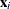, is independently Poisson-distributed as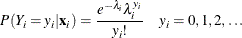The Conway-Maxwell-Poisson distribution is defined as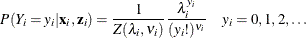where the normalization factor is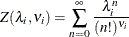and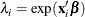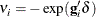Thevector is a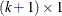parameter vector. (The intercept is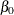, and the coefficients for the k regressors are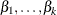). The
vector is an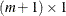parameter vector. (The intercept is represented by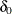, and the coefficients for the m regressors are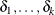). The covariates are represented by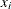and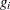vectors.

One of the restrictive properties of the Poisson model is that the conditional mean and variance must be equal: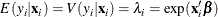The CMP distribution overcomes this restriction by defining an additional parameter,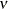, which governs the rate of decay of successive ratios of probabilities such that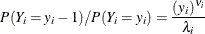The introduction of the additional parameter,, allows for flexibility in modeling the tail behavior of the distribution. If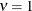, the ratio is equal to the rate of decay of the Poisson distribution. If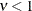, the rate of decay decreases, enabling you to model processes that have longer tails than the Poisson distribution (overdispersed data). If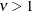, the rate of decay increases in a nonlinear fashion, thus shortening the tail of the distribution (underdispersed data).

There are several special cases of the Conway-Maxwell-Poisson distribution. If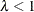and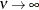, the Conway-Maxwell-Poisson results in the Bernoulli distribution. In this case, the data can take only the values 0 and 1, which represents an extreme underdispersion. If, the Poisson distribution is recovered with its equidispersion property. When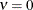and, the normalization factor is convergent and forms a geometric series,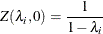and the probability density function becomes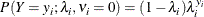The geometric distribution represents a case of severe overdispersion.

Mean, Variance, and Dispersion for the Conway-Maxwell-Poisson Model

The mean and the variance of the Conway-Maxwell-Poisson distribution are defined as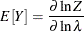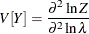The Conway-Maxwell-Poisson distribution does not have closed-form expressions for its moments in terms of its parametersand. However, the moments can be approximated. Shmueli et al. (2005) use asymptotic expressions for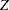to derive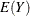and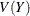as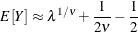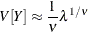In the Conway-Maxwell-Poisson model, the summation of infinite series is evaluated using a logarithmic expansion. The mean and variance are calculated as follows for the Shmueli et al. (2005) model: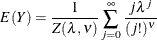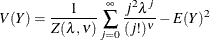The dispersion is defined as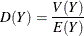Likelihood Function for the Conway-Maxwell-Poisson Model

The likelihood for a set of n independently and identically distributed variables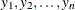is written as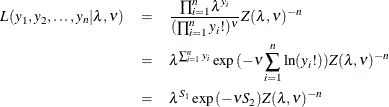where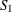and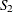are sufficient statistics for. You can see from the preceding equation that the Conway-Maxwell-Poisson distribution is a member of the exponential family. The log-likelihood function can be written as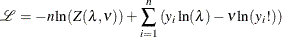The gradients can be written as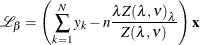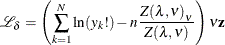Conway-Maxwell-Poisson Regression: Guikema and Coffelt (2008) Reparameterization

Guikema and Coffelt (2008) propose a reparameterization of the Shmueli et al. (2005) Conway-Maxwell-Poisson model to provide a measure of central tendency that can be interpreted in the context of the generalized linear model. By substituting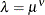, the Guikema and Coffelt (2008) formulation is written as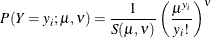where the new normalization factor is defined as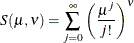In terms of their new formulations, the mean and variance of Y are given as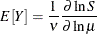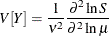They can be approximated as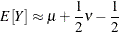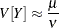In the COUNTREG procedure, the mean and variance are calculated according to the following formulas for the Guikema and Coffelt (2008) model: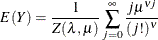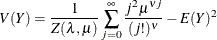In terms of the new parameter, the log-likelihood function is specified as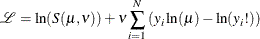and the gradients are calculated as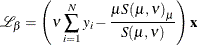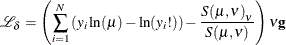The default in the COUNTREG procedure is the Guikema and Coffelt (2008) specification. The Shmueli et al. (2005) model can be estimated by specifying the PARAMETER=LAMBDA option. If you specify DISP=CMPOISSON in the MODEL statement and you omit the DISPMODEL statement, the model is estimated according to the Lord, Guikema, and Geedipally (2008) specification, whererepresents a single parameter that does not depend on any covariates. The Lord, Guikema, and Geedipally (2008) specification makes the model comparable to the negative binomial model because it has only one parameter.

The dispersion is defined asUsing the Guikema and Coffelt (2008) specification results in the integral part ofrepresenting the mode, which is a reasonable approximation for the mean. The dispersion can be written as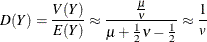When< 1, the variance can be shown to be greater than the mean and the dispersion greater than 1. This is a result of overdispersed data. When= 1, and the mean and variance are equal, the dispersion is equal to 1 (Poisson model). When> 1, the variance is smaller than the mean and the dispersion is less than 1. This is a result of underdispersed data.

All Conway-Maxwell-Poisson models in the COUNTREG procedure are parameterized in terms of dispersion, where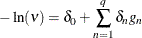Negative values of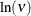indicate that the data are approximately underdispersed, and values ofthat are greater than 0 indicate that the data are approximately overdispersed.